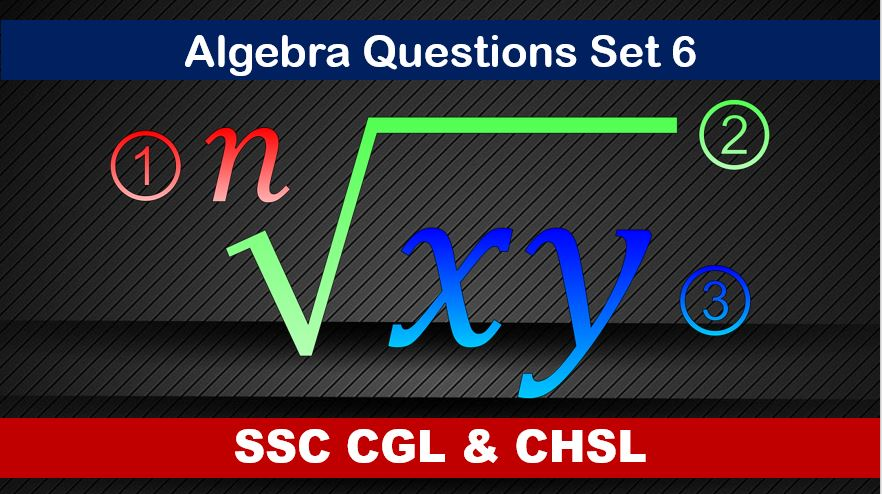Monday, September 27, 2021
Home > algebra > SSC CHSL Algebra Questions and Solutions Set 6

# SSC CHSL Algebra Questions and Solutions Set 6SSC CGL CHSL Algebra
Algebra Questions Sets
Set 1Set 2Set 3Set 4Set 5
Set 6

SSC CHSL Algebra Questions and Solutions Set 6 : Ques No 1

If a2 + b2 = 2 and c2 + d2 = 1, then the value of (ad – bc)2 + (ac + bd)2 is

Options:

A. 4/9

B. 1/2

C. 1

D. 2

Solution:

SSC CHSL Algebra Questions and Solutions Set 6 : Ques No 2

If xa.xb.xc = 1, then the value of a3 + b3 + c3 is

Options:

A. 9

B. abc

C. a + b + c

D. 3abc

Solution:

SSC CHSL Algebra Questions and Solutions Set 6 : Ques No 3

If 2x = √a + 1/√a, a > 0, then the value of √(x2 – 1) / (x – √(x2 – 1)) is

Options:

A. a + 1

B. (1/2)(a – 1)

C. a – 1

D. (1/2)(a + 1)

Solution:

SSC CHSL Algebra Questions and Solutions Set 6 : Ques No 4

If x + 2/x = 1, then the value of (x2 + x + 2) / (x2(1 – x)) is

Options:

A. 2

B. -2

C. 1

D. -1

Solution:

SSC CHSL Algebra Questions and Solutions Set 6 : Ques No 5

If a3 – b3 – c3 = 0, then the value of a9 – b9 – c9 – 3a3b3c3 is

Options:

A. 1

B. 2

C. 0

D. -1

Solution:

SSC CHSL Algebra Questions and Solutions Set 6 : Ques No 6

If a + 1/a = 2, then the value of (5a2 + 5) / (3a2 + 9a + 3) is

Options:

A. 2/3

B. 0

C. 1/3

D. 1

Solution:

SSC CHSL Algebra Questions and Solutions Set 6 : Ques No 7

If P2 + 1/P2 = 1 (P > 0), then the value of P3 + 1/P3 is

Options:

A. 1

B. 2

C. 3

D. 0

Solution:

SSC CHSL Algebra Questions and Solutions Set 6 : Ques No 8

If a = 1, b = 2 and c = -3, then the value of (a3 + b3 + c3 – 3abc) / (ab + bc + ca – (a2 + b2 + c2)) is

Options:

A. 3

B. 2

C. 0

D. 1

Solution:

SSC CHSL Algebra Questions and Solutions Set 6 : Ques No 9

If a – b = 2, ab = 15, then the value of (a2 – b2)(a3 – b3) is

Options:

A. 1450

B. 1500

C. 1528

D. 1568

Solution:

SSC CHSL Algebra Questions and Solutions Set 6 : Ques No 10

If xy/(x + y) = a, xz/(x + z) = b and yz/(y + z) = c, then the value of x is

Options:

A. abc/(ab + bc + ca)

B. 2abc/(ab + bc – ca)

C. 2abc/(ab + ac – bc)

D. 2abc/(ac + bc – ab)

Solution:

SSC CHSL Algebra Questions and Solutions Set 6 : Ques No 11

The value of (1 + 1/x)(1 + 1/(x + 1))(1 + 1/(x + 2))(1 + 1/(x + 3)) is

Options:

A. (1 + 1/(x + 4))

B. (x + 4)

C. 1/x

D. (x + 4)/x

SSC CHSL Algebra Questions and Solutions Set 6 : Ques No 12

If 2p/(p2 – 2p + 1) = 1/4, then p0, then the value of p + 1/p is

Options:

A. 4

B. 5

C. 10

D. 12

SSC CHSL Algebra Questions and Solutions Set 6 : Ques No 13

If, then the value of x is

Options:

A. 31

B. 32

C. 36

D. 37

SSC CHSL Algebra Questions and Solutions Set 6 : Ques No 14

If a/(1-a) + b/(1-b) + c/(1-c) = 1, then the value of 1/(1-a) + 1/(1-b) + 1/(1-c) is

Options:

A. 1

B. 2

C. 3

D. 4

SSC CHSL Algebra Questions and Solutions Set 6 : Ques No 14

If x varies inversely as (y2 – 1) and is equal to 24 when y = 10, then the value of x when y = 5 is

Options:

A. 99

B. 12

C. 24

D. 100